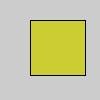Name

<< (left shift)

Examples
m = 1 << 3  # In binary: 1 to 1000
print(m)  # Prints "8"
n = 1 << 8  # In binary: 1 to 100000000
print(n)  # Prints "256"
o = 2 << 3  # In binary: 10 to 10000
print(o)  # Prints "16"
p = 13 << 1 # In binary: 1101 to 11010
print(p)  # Prints "26"# Packs four 8 bit numbers into one 32 bit number
a = 255     # Binary: 00000000000000000000000011111111
r = 204     # Binary: 00000000000000000000000011001100
g = 204     # Binary: 00000000000000000000000011001100
b = 51      # Binary: 00000000000000000000000000110011
a = a << 24 # Binary: 11111111000000000000000000000000
r = r << 16 # Binary: 00000000110011000000000000000000
g = g << 8  # Binary: 00000000000000001100110000000000
# Equivalent to "color argb = color(r, g, b, a)" but faster
argb = a | r | g | b
fill(argb)
rect(30, 20, 55, 55)
Description Shifts bits to the left. The number to the left of the operator is shifted the number of places specified by the number to the right. Each shift to the left doubles the number, therefore each left shift multiplies the original number by 2. Use the left shift for fast multiplication or to pack a group of numbers together into one larger number. Left shifting only works with integers or numbers which automatically convert to an integer such as byte.
Syntax
value << n
Parameters
value int: the value to shift int: the number of places to shift left
Related >> (right shift)

Updated on Mon Sep 21 15:53:25 2020.

If you see any errors or have comments, please let us know.